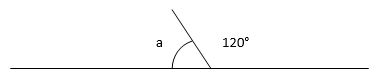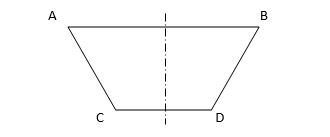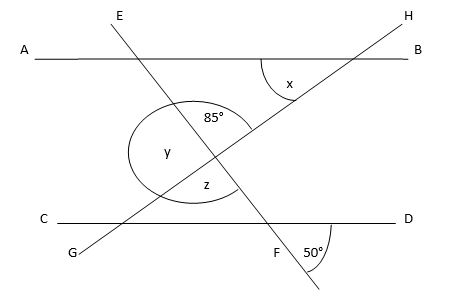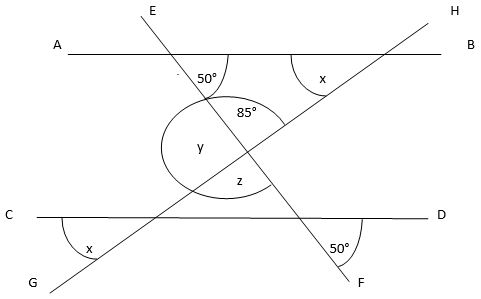# Finding Missing Angles in Shapes and Finding Angles Outside of Shapes

Finding Missing Angles in Shapes

Often children will be presented with a shape and be expected to estimate what an angle is. If they are familiar with the basics of a protractor they should be able to cope with this. However, often they will need to know a precise angle on a shape on which the other angles are given.

If it is a straight line:A straight line has a total of 180° in it, so angle a must be 180° take away the part of the angle that we already know (i.e. 120°). Angle a must be 180-120 = 60°.

Similarly, if the figure is a triangle and one angle is missing, the total of the angles (180° again) minus the two angles that are given, must equal the unknown angle.

If the triangle has angles of 55°, 90° and n, then we find what n is by taking 55° and 90° from 180°, leaving 35°.

The same applies to a quadrilateral, but of course the total degrees in a four sided shape isn't 180° but 360°.If we know that a certain shape is being used, for instance the one above we are told is symmetrical, we will know that the top two angles will be the same and the bottom two angles will be the same. If we are told that angle A is 61° then we know angle B is 61° as well.

That leaves (360° - 61° - 61° = ) 238° which must be the total of angles C and D. Given they must be the same as each other, they are 238° ÷ 2 = 119° each.

Finding Angles Outside of Shapes

This is a skill that I find a lot of children don't have but it's one which is very easy once you develop a good sense of visualising shapes and angles, and once you know the quite simple rules that govern them. Although there are an incredible number of devious possibilities of how a question can be set up, it will probably boil down to a handful of possible rules that will explain it.

Firstly, you need to know the rules of finding missing angles within shapes. Knowing that there are 180° in a triangle, that an isosceles triangle has two identical angles, that a quadrilateral has 360° in it... these are taken as read by the examination board. They will then set up questions that utilise this knowledge like this:

Line AB is parallel to line CD. What are the values of angles x, y and z?It is important to note that the lines AB and CD are parallel - this means that any single line which cuts through one of them will do so at the same angle as the other. In other words, we can tell the angles of things which are apparently not possible to spot! On our diagram, I can now use that knowledge to show which other angles are similar to the ones we know or are being asked for. Note that we know that the second angle of the top triangle, where line AB meets line EF, is 50° as it is identical to the angle made at the intersection of line CD meeting line EF. That's because AB and CD are parallel.Now we can work out angle x as it is the third angle of a triangle. 180° - 85° - 50° = 45°

The second useful bit of knowledge is that opposite angles are identical. Look at the intersection of the lines at the centre of the diagram. Where they meet, the angles opposite each other are going to be the same - so angle z must be 85°.

Angle y is worked out by saying that it, along with 85°, makes a straight line. As we know a straight line is 180°, then angle y is (180° - 85° = ) 95°.

From just two angles and knowledge of parallel lines and opposite angles, we've found the angles which at first seemed impossible to work out.